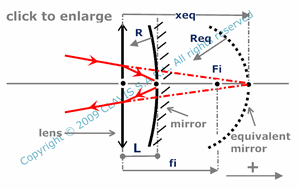and orgeometrical optics
focal lengths and principal planes

# combination of a thin lens and a spherical mirror

Caution
Fill in all input cells.
Choose all parameters unit.
Except L which is positive, distances and raddii are algebric.
Positive direction is according the horizontal arrow in the bottom right of the scheme.
As light rays pass through the lens twice ( before and after reflection on the mirror ), fi sign can be confuzing. Sign is positive for converging lens and negative for diverging lens.

InputValueunit
R
fi
L
OutputValueUnit
xeqto be calculated
Reqto be calculatedR : radius of curvature of the mirror fi : effective focal length of the lens L : distance between the lens and the mirror vertex xeq : distance from the lens to the vertex of the equivalent mirror Req : radius of curvature of the equivalent mirror

Note
This calculation page can not detect all unrealistic inputs.